﻿ FPSB与连接浮桥耦合运动响应分析
 舰船科学技术2019, Vol. 41Issue (3): 112-117PDF
FPSB与连接浮桥耦合运动响应分析

Research on coupled motion response of fpsb with the pontoon bridge
YAN Liu, LIAN Xue-hai, WANG Xiao-chun, MU Jian-yi, CHEN Dai-dai
CETC Maritime Electronics Research Institute Co., Ltd., Ningbo 315000, China
Abstract: As a new type of floating platform for reef development, FPSB (Floating Production Storage Bridge) is connected to the offshore reefs by the floating pontoon bridge. However, the existing norms are lack of theoretical methods for the calculation of the effect of the pontoon bridge on the ship motion response. Based on 3D linear hydrodynamic, the six-degree quasi-static coupling equation of the FPSB and the pontoon bridge is established. The code is developed to calculate the motion and the load responses of the FPSB with the influence of the pontoon bridge. Then the response of the motion and wave load of the hull is analyzed and compared before and after the impact of the pontoon bridge is considered under the typical load conditions. The numerical results show that the pontoon bridge has a greater impact on the heave and pitching of the hull. The amplitude of the vertical wave bending moment and the vertical wave shear force tends to be generally larger after taking into account the influence of the pontoon bridge. The research of this paper can be a reference to the calculation of the coupled motion response of the ship and pontoon bridge.
0 引　言

1 三维源汇分布法

 $\varPhi (x,y,z,t) = \phi (x,y,z){e^{ - i\omega t}}\text{，}$ (1)

 $\phi (x,y,z){e^{ - i\omega t}} = \left[ {\left( {{\phi _I} + {\phi _d}} \right) + \sum\limits_{j = 1}^6 {{\varphi _j}{x_j}} } \right]{e^{ - i\omega t}}\text{。}$ (2)

${\phi _j}$ 满足如下边界条件：

 $p = - \rho \frac{{\partial \Phi }}{{\partial t}}\text{；}$ (3)

 ${{F}} = - i\rho \omega {e^{ - i\omega }}\iint\nolimits_{{S_B}} {\left( {{\phi _I} + {\phi _D}} \right){{n}}{\rm d}S}\text{。}$ (4)

 ${F_{ji}} = - \rho {\omega ^2}\iint\nolimits_{{S_B}} {{\phi _i}{n_j}{\rm d}S},\;\;\;\;\;i,j = 1,2,\cdots,6\text{，}$ (5)

 ${F_{ji}} = {\rm{ - }}{A_{ji}}{\ddot x_i} - {B_{ji}}{\dot x_i}\text{。}$ (6)

 $F\left( \omega \right) = {F_j} + {F_{ji}}\text{。}$ (7)

2 FPSB与浮桥耦合运动方程的建立

FPSB通过浮筒桁架式浮桥与岛礁连接，如图1所示，主船体与浮桥之间采用A字型钢架连接，如图2所示。与传统的锚链连接方式不同，该连接方式约束了2个浮体之间的线位移而不约束角位移，使得钢架能够传递力而不传递弯矩，因而浮桥能够与FPSB主船体的运动保持同频率同相位，方便建立主船体和连接浮桥的耦合水动力模型。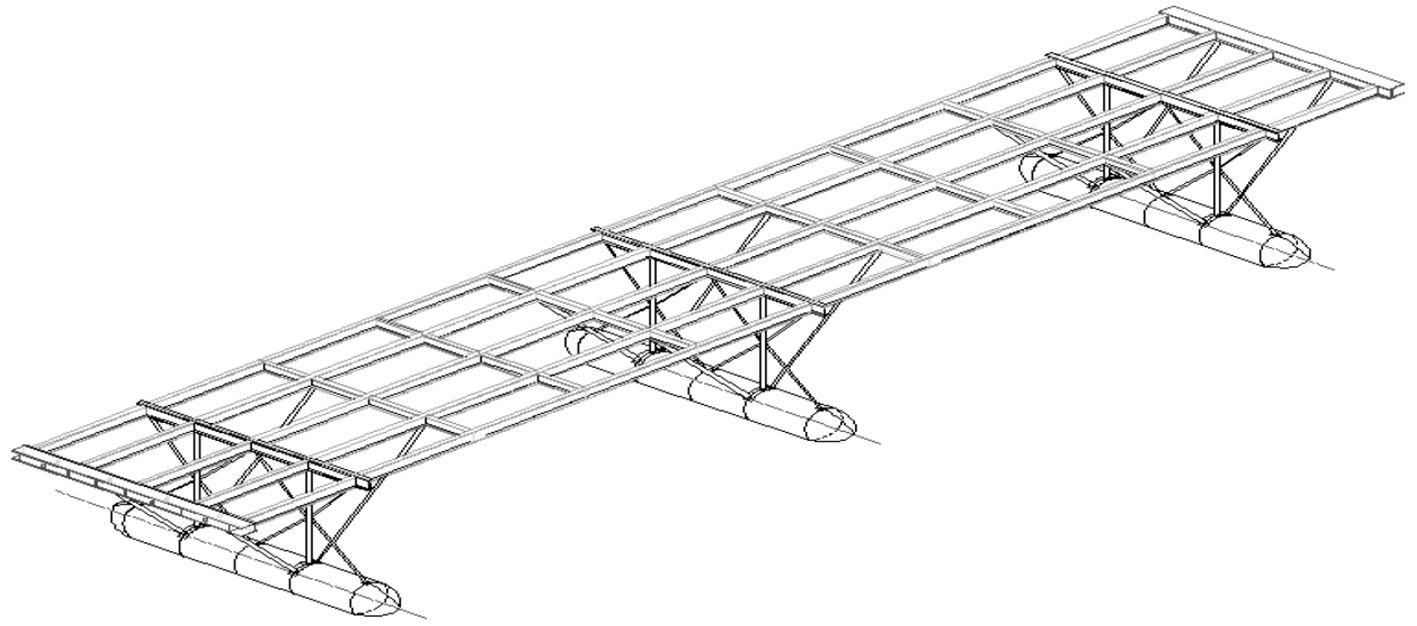图 1 连接浮桥示意图 Fig. 1 The sketch of pontoon bridge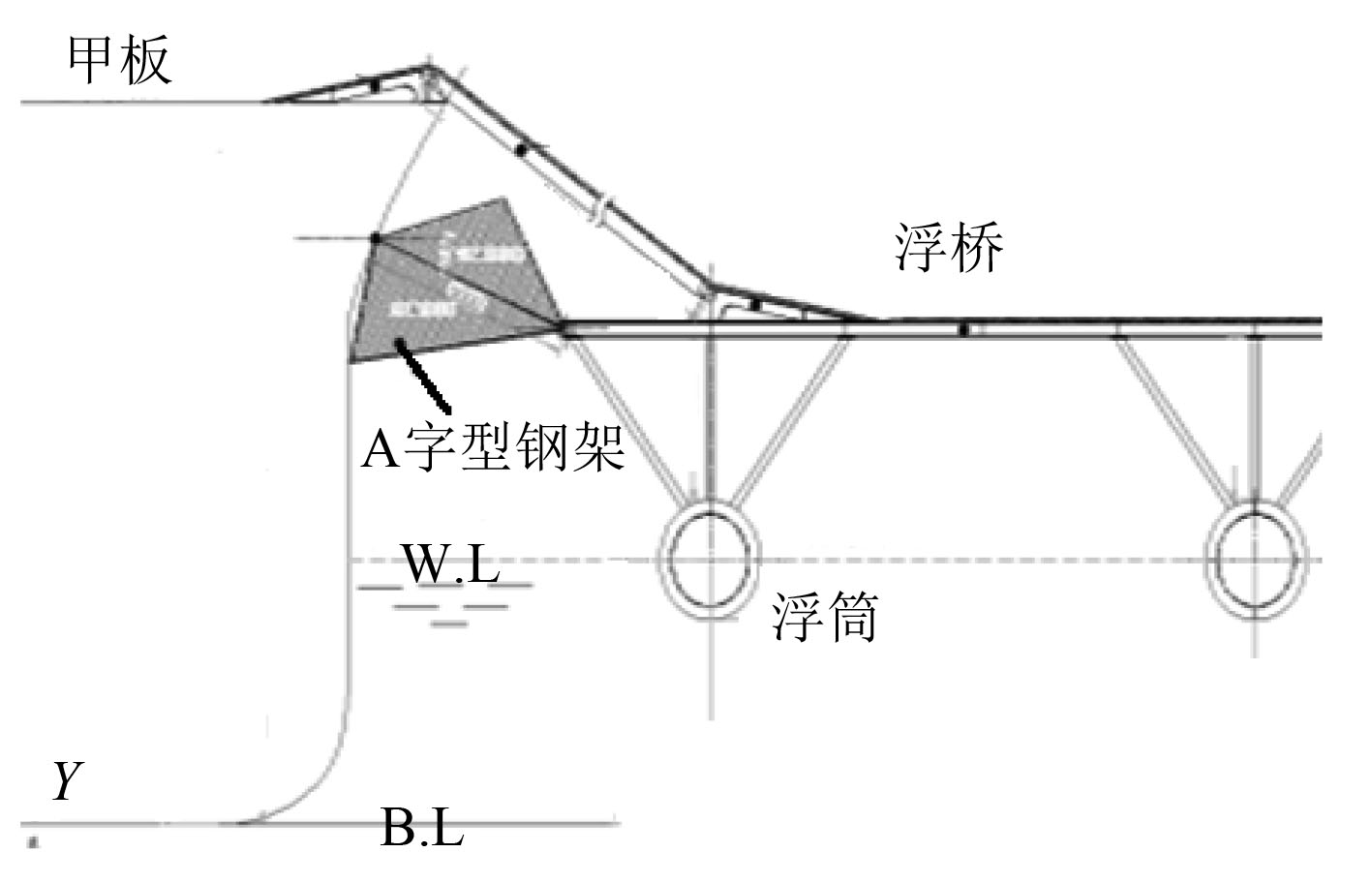图 2 A字型钢架示意图 Fig. 2 The sketch of A type steel frame

 $\left( { { M} + { A} } \right)\left\{ {\ddot \eta (t)} \right\} + { B} \left\{ {\dot \eta (t)} \right\} + { C} \left\{ {\eta (t)} \right\} = {f_{wave}} + {f_{\!F\!P\!S\!B}}\text{，}$ (8)

 $\begin{split} & \left( { {{ M}'} + {{ A}'} } \right)\left\{ {\ddot \eta (t)} \right\} + {{ B}'} \left\{ {\dot \eta (t)} \right\} + {{ C}'} \left\{ {\eta (t)} \right\} = \\ & {F_{wave}} + {F_{\text{浮桥}}}\text{。} \end{split}$ (9)

 ${f_{FPSB}} + {F_{\text{浮桥}}} = 0\text{。}$ (10)

 $\begin{split} & \left( { { M} + { A} + {{ M}'} + {{ A}'} } \right)\left\{ {\ddot \eta (t)} \right\} + \left[ {{ B} + { B}'} \right]\left\{ {\dot \eta (t)} \right\} +\\ & \left[ {{ C} + { C}'} \right]\left\{ {\eta (t)} \right\} = {f_{wave}} + {F_{wave}}\text{。} \end{split}$ (11)
3 运动与载荷的数值计算 3.1 水动力模型的建立表 1 FPSB和浮桥主要设计参数 Tab.1 Main characteristic parameters of the FPSB and pontoon bridge

FPSB主船体和连接浮桥的水动力模型分别如图3图4所示。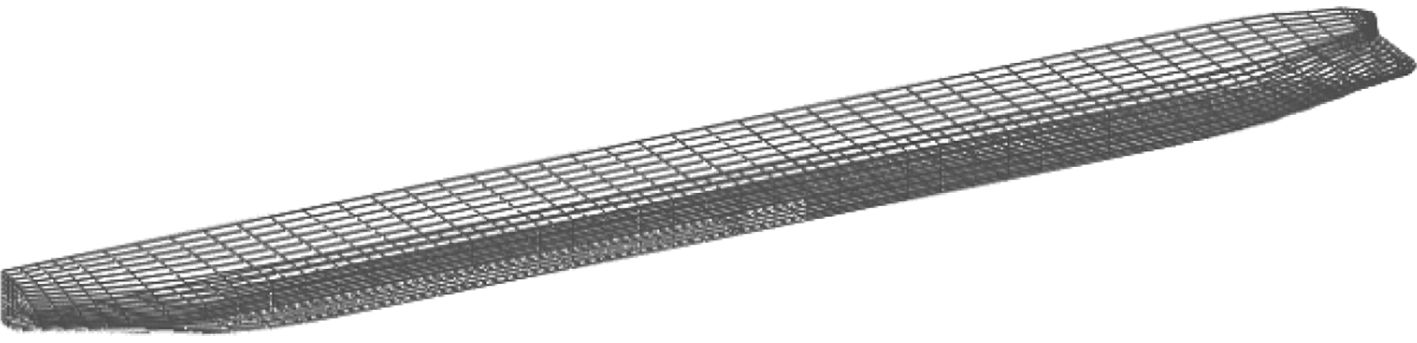图 3 FPSB水动力模型 Fig. 3 The hydrodynamic model of FPSB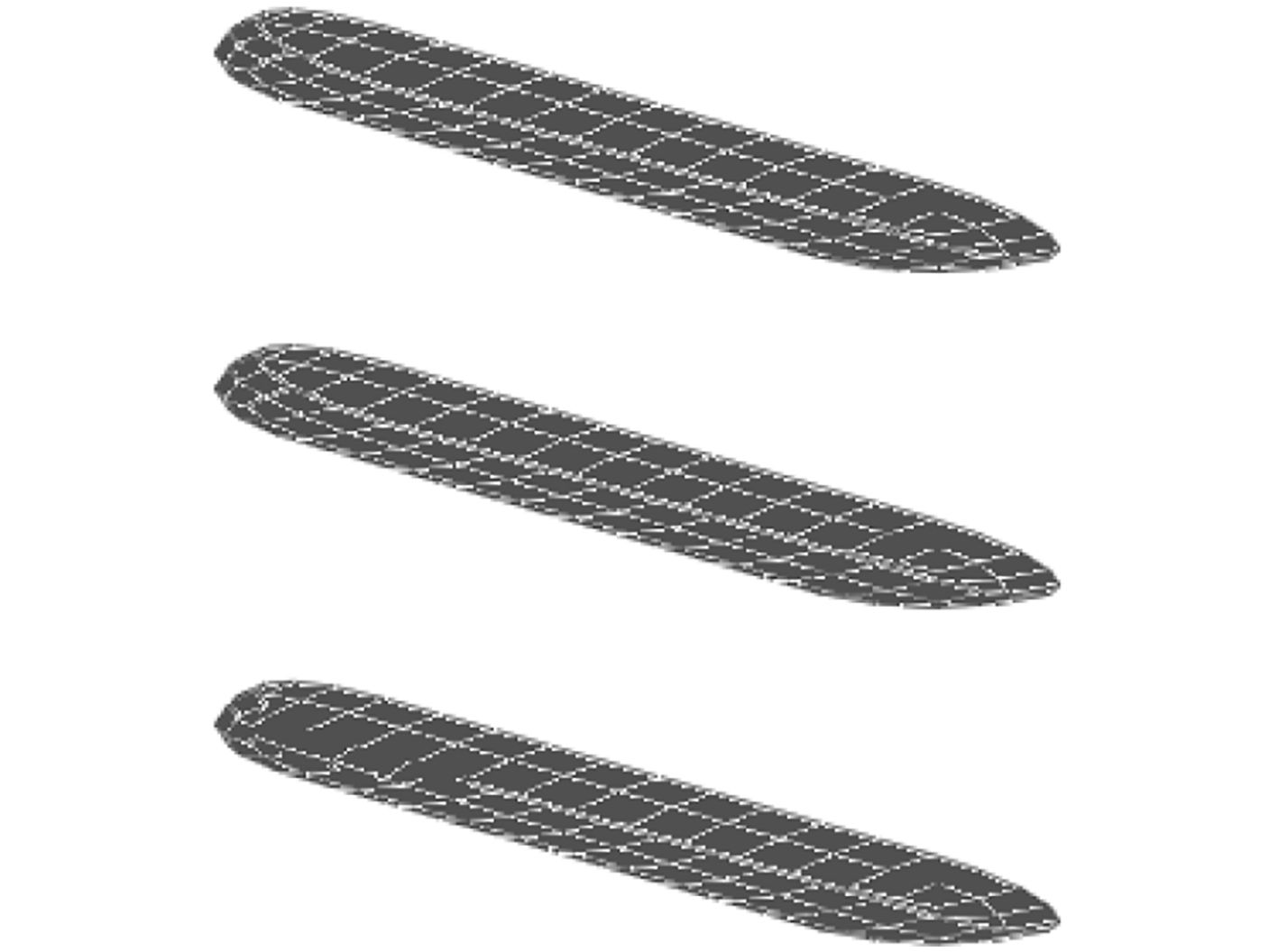图 4 浮筒水动力模型 Fig. 4 The hydrodynamic model of the buoy
3.2 运动响应分析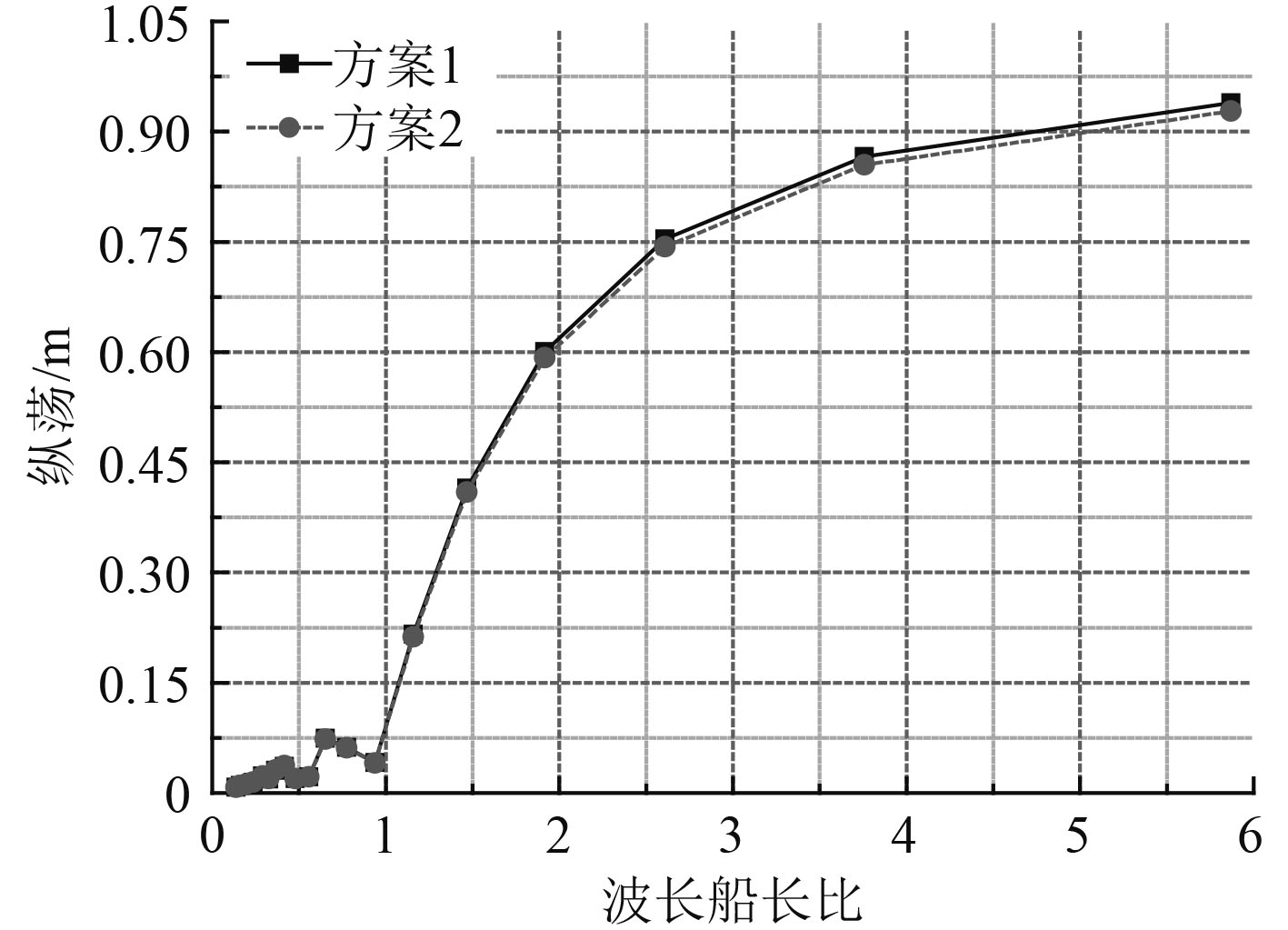图 5 纵荡幅值对比 Fig. 5 Comparison of surge amplitude response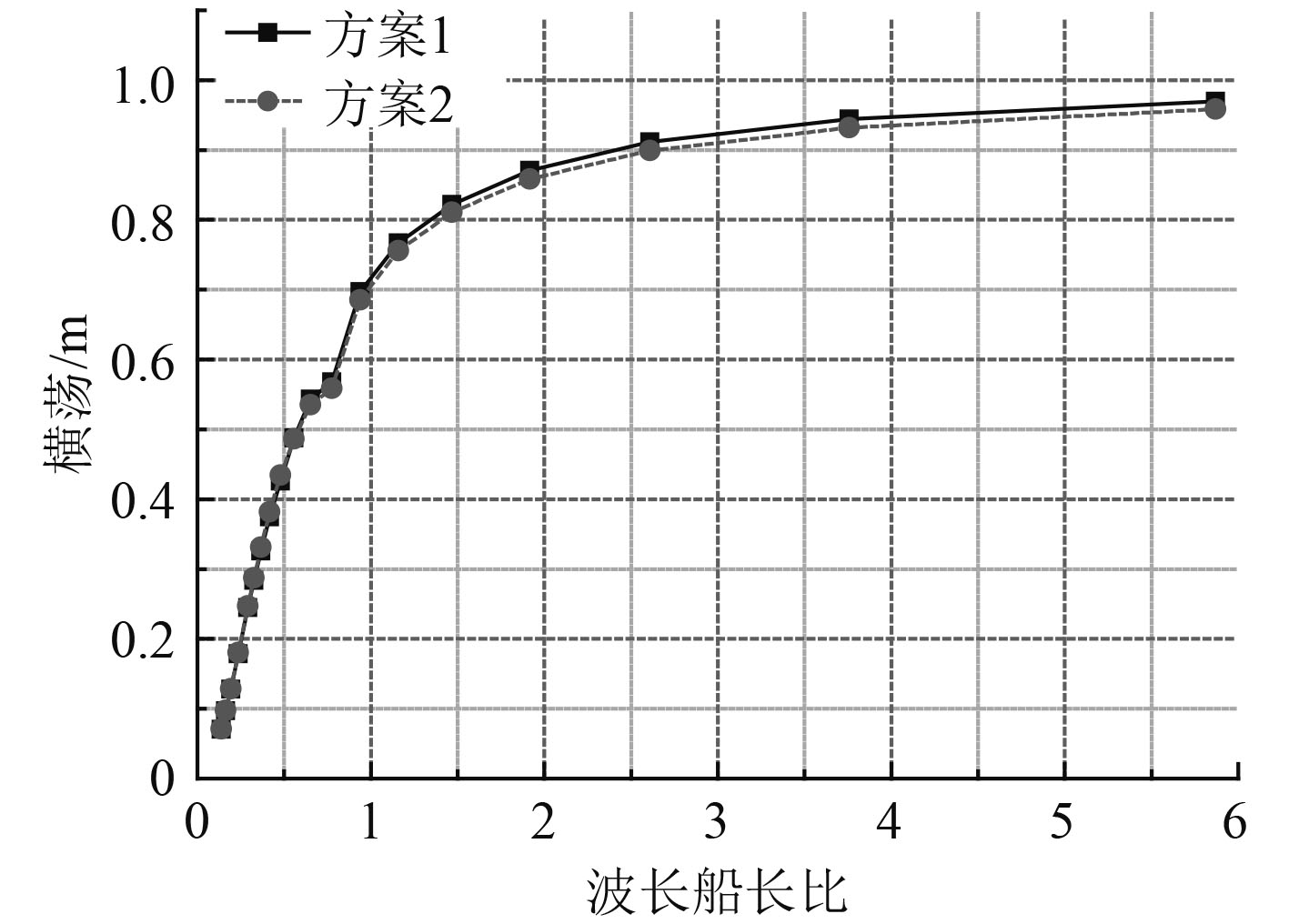图 6 横荡幅值对比 Fig. 6 Comparison of sway amplitude response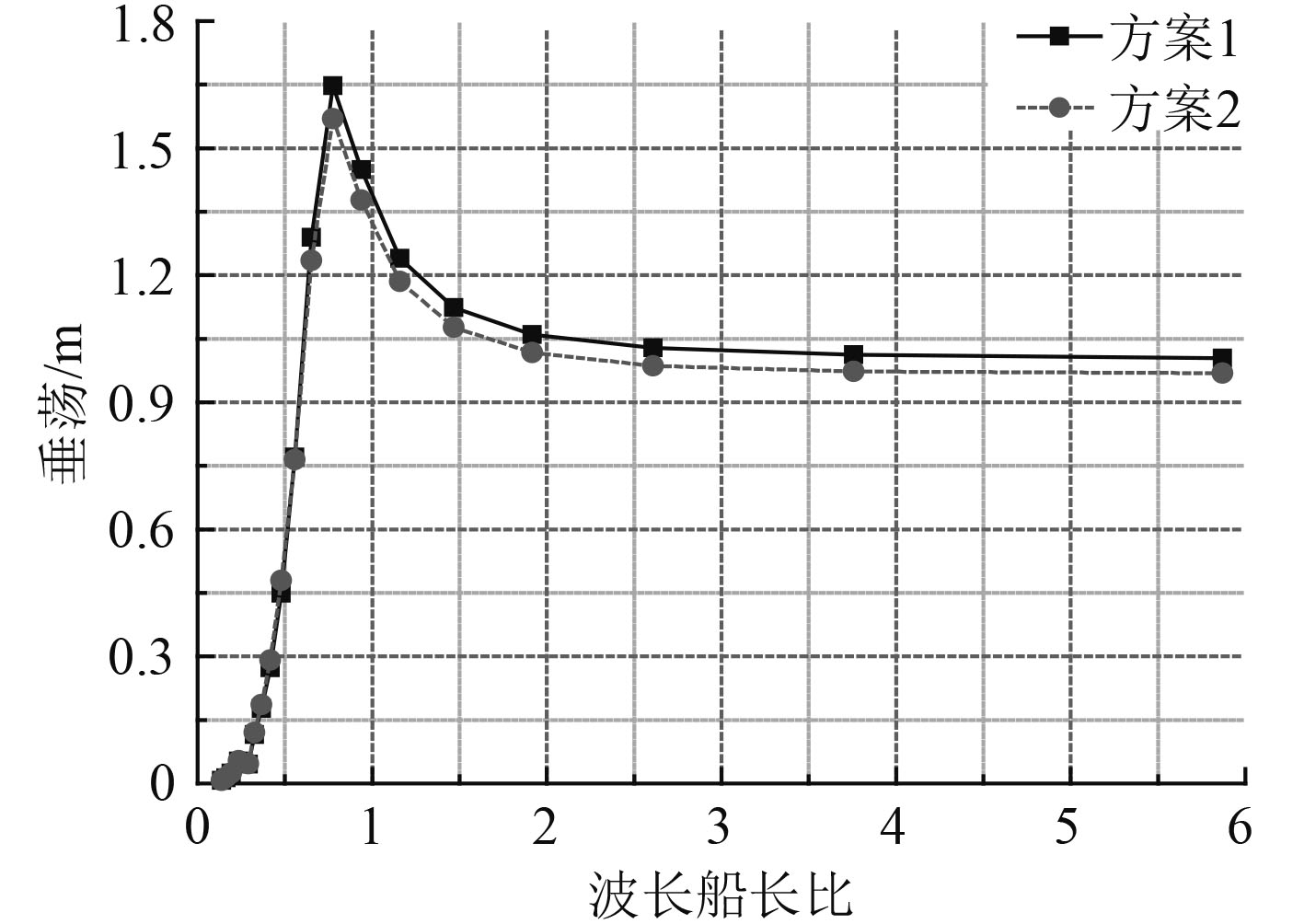图 7 垂荡幅值对比 Fig. 7 Comparison of heave amplitude response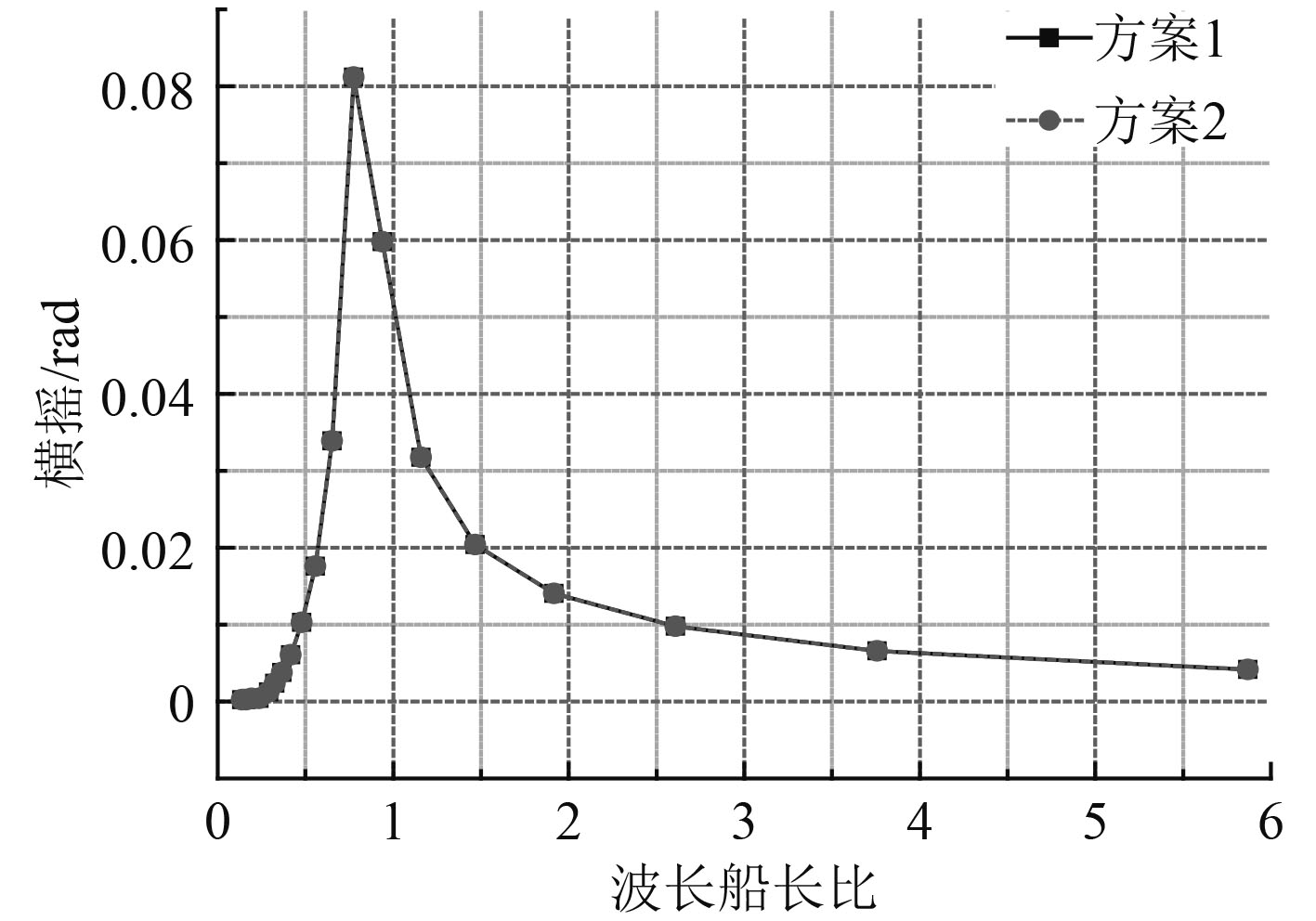图 8 横摇幅值对比 Fig. 8 Comparison of roll amplitude response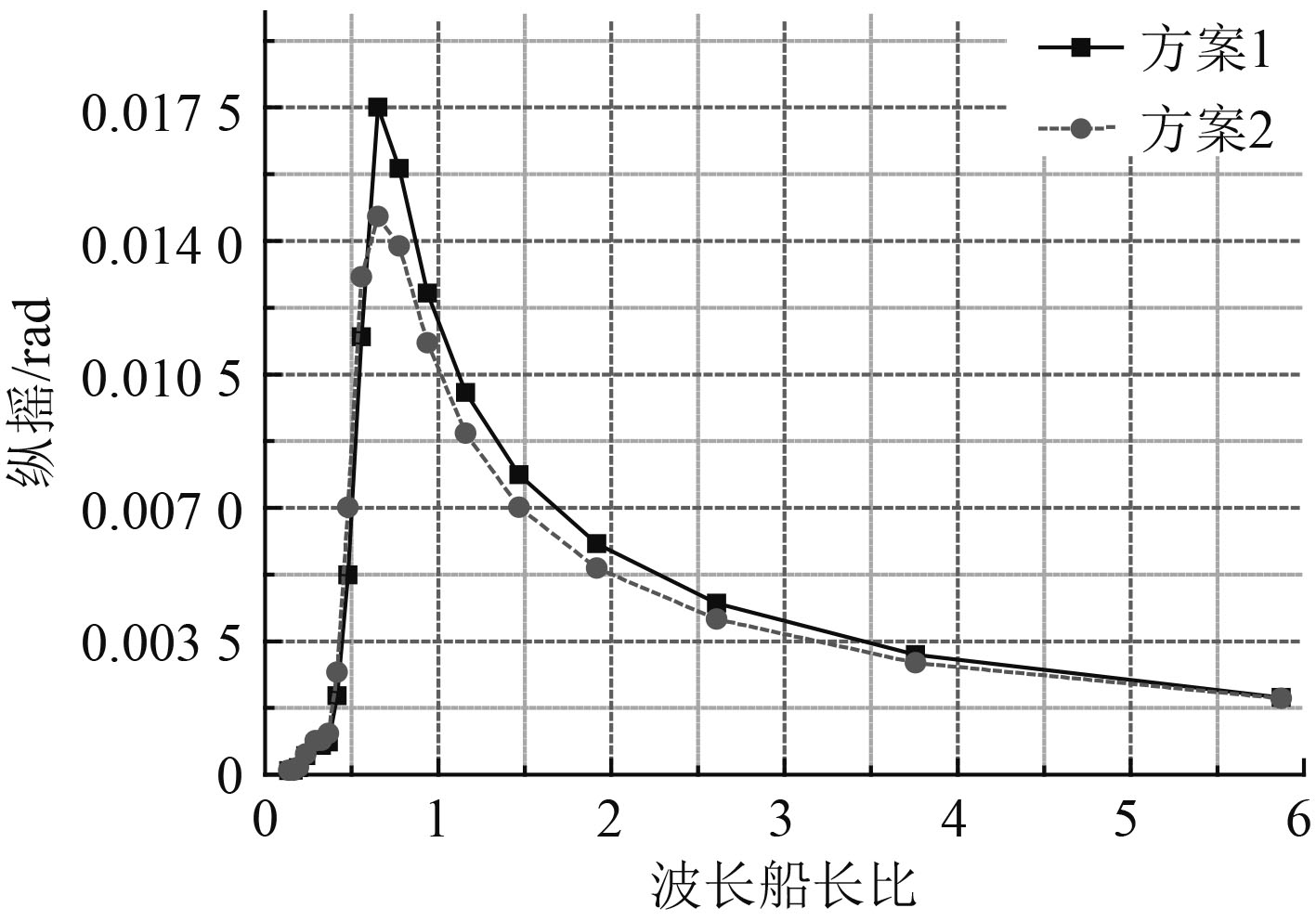图 9 纵摇幅值对比 Fig. 9 Comparison of pitch amplitude response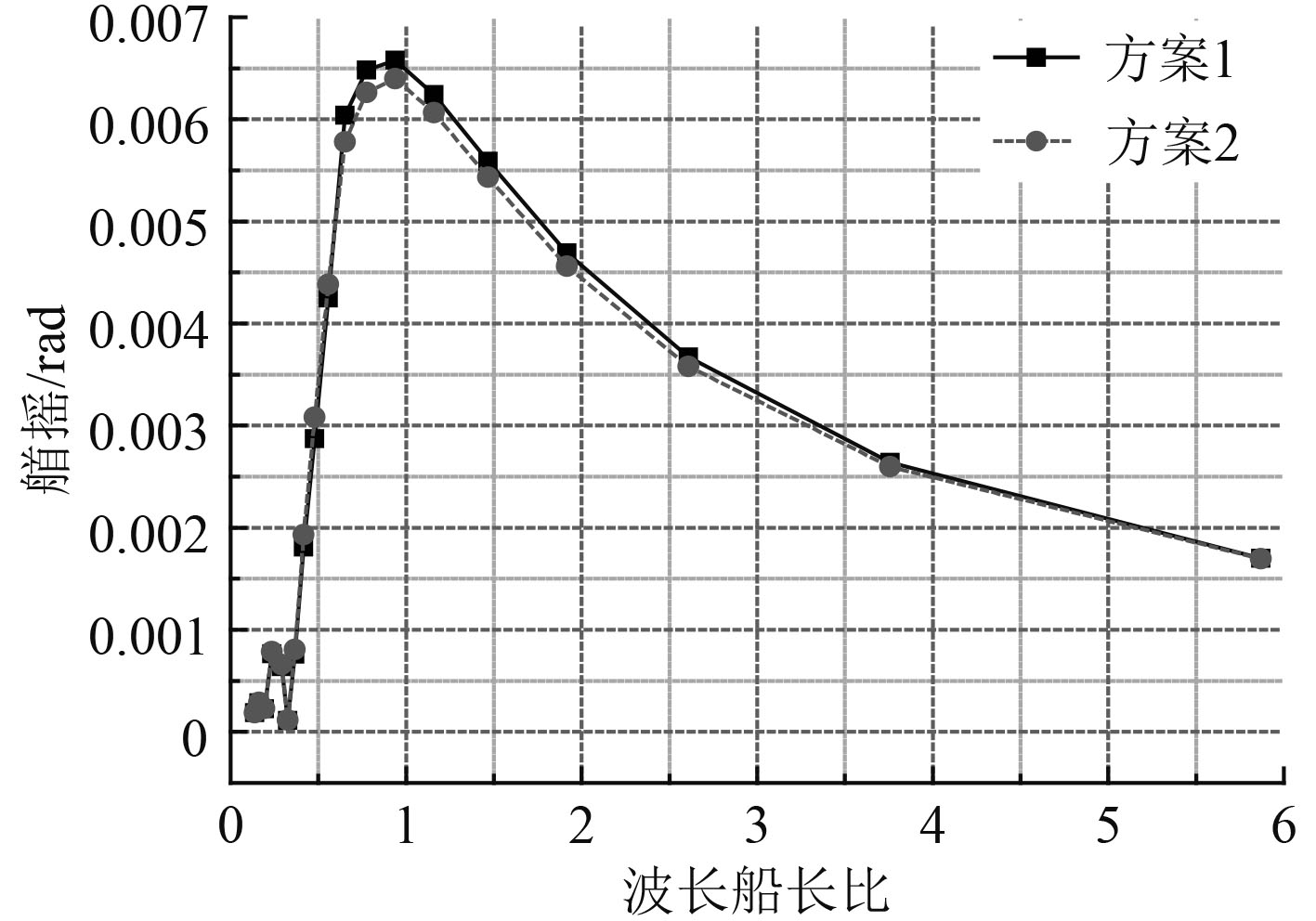图 10 艏摇幅值对比 Fig. 10 Comparison of yaw amplitude response表 2 满载工况下六自由度运动幅值对比 Tab.2 Comparison of motion amplitude of six-degree between caseI and caseII on full load condition

3.3 载荷响应分析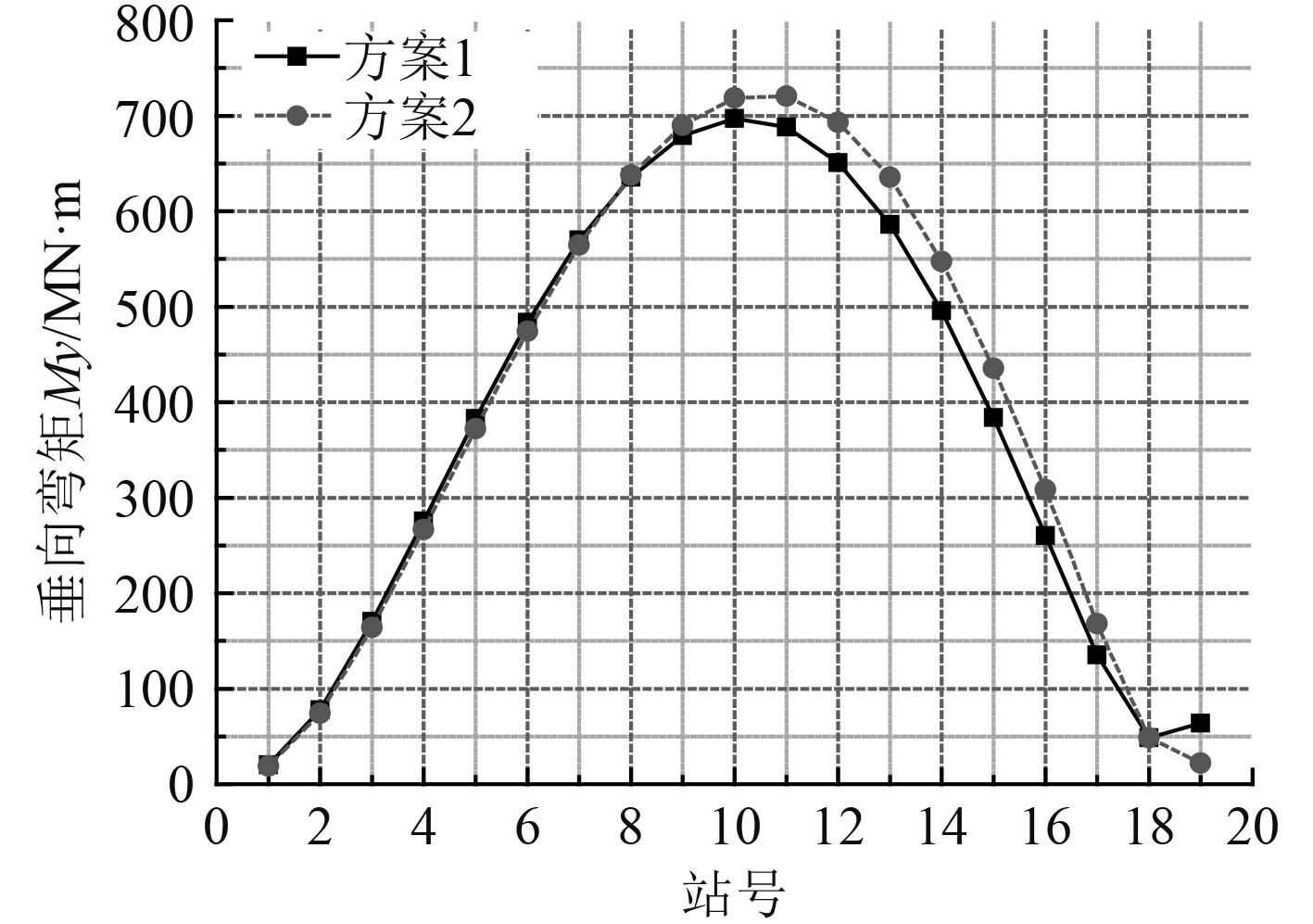图 11 垂向弯矩响应对比 Fig. 11 Comparison of the vertical bending moment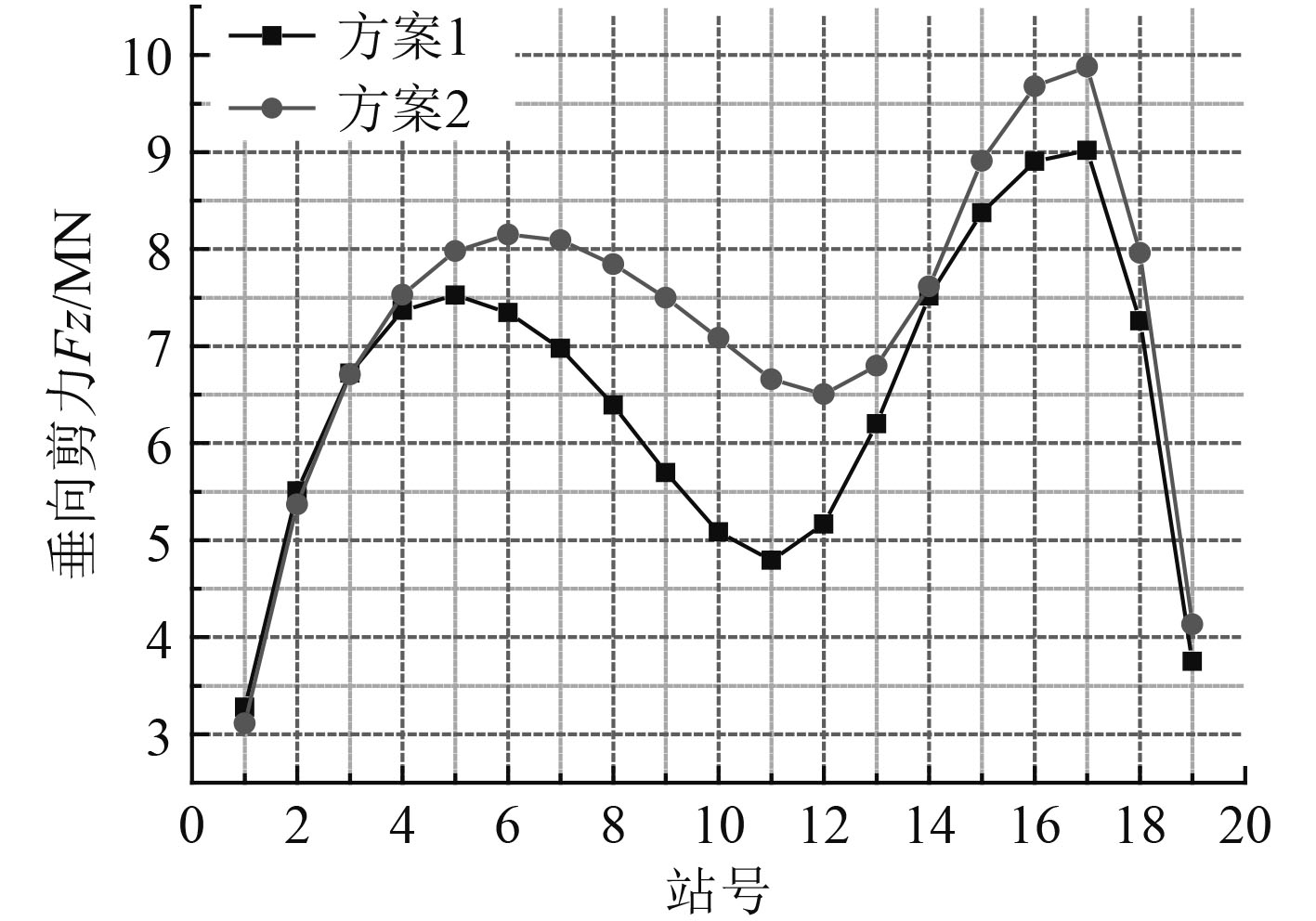图 12 垂向剪力响应对比 Fig. 12 Comparison of the vertical shear force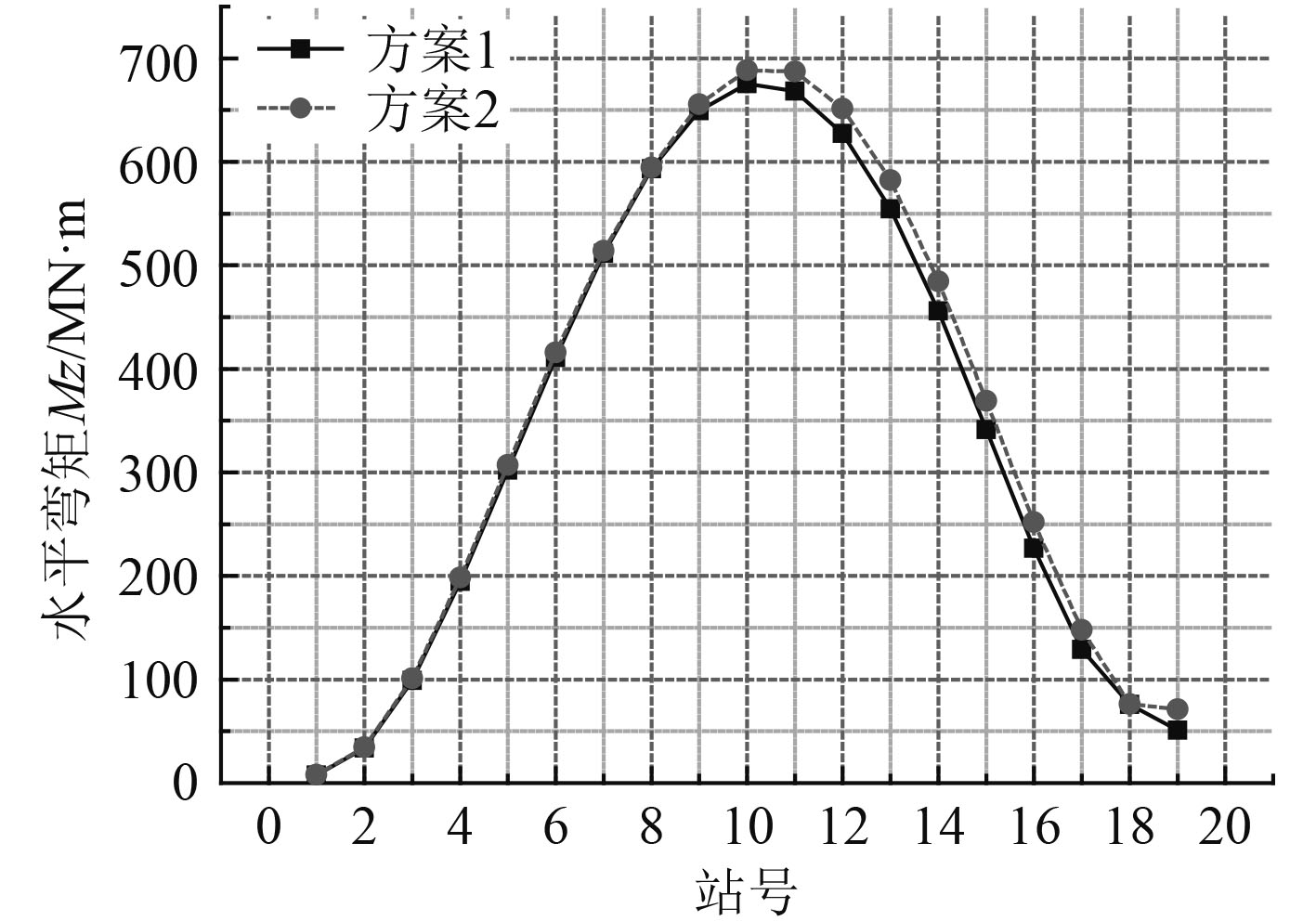图 13 水平弯矩响应对比 Fig. 13 Comparison of the horizontal bending moment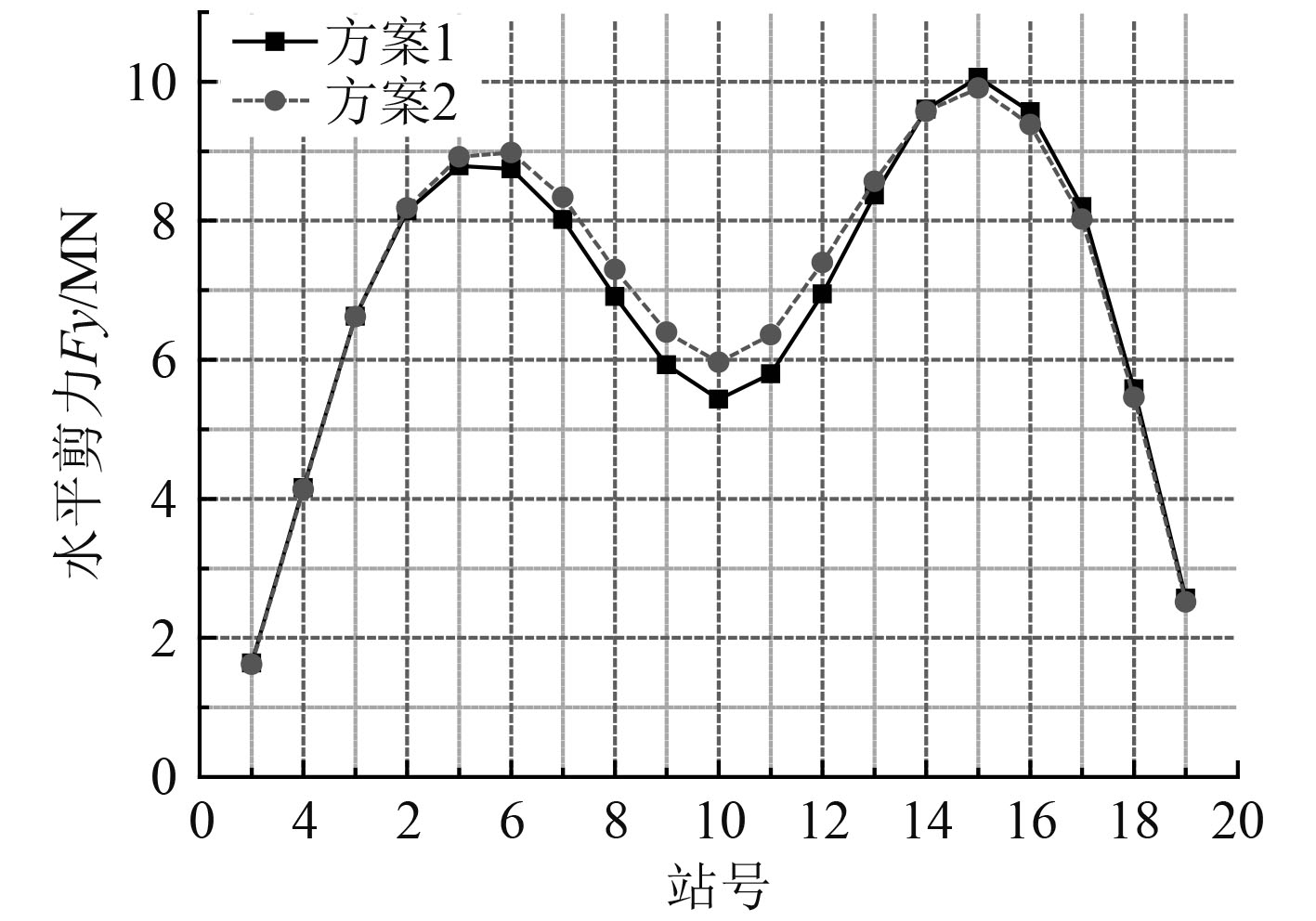图 14 水平剪力响应对比 Fig. 14 Comparison of the horizontal shear force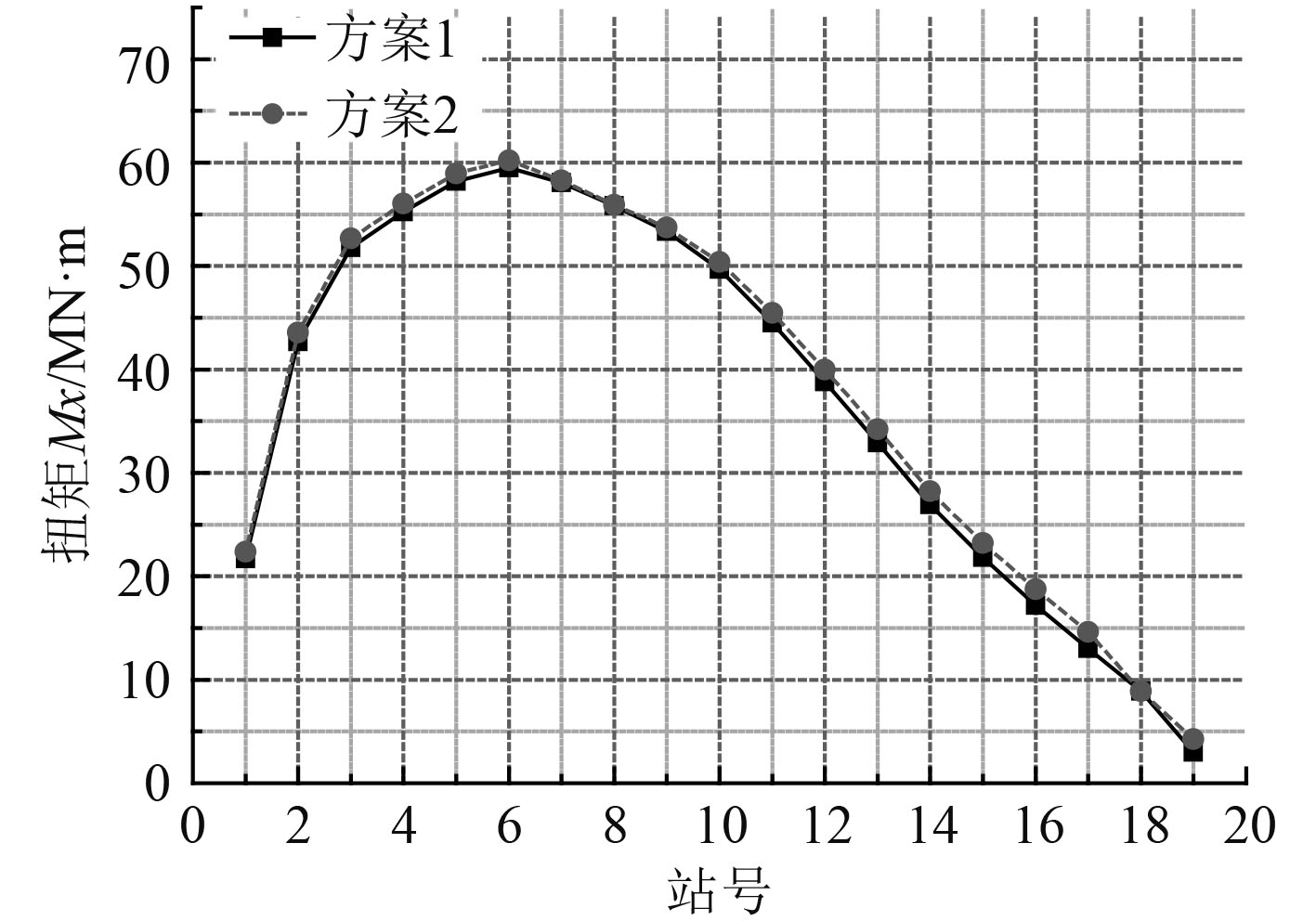图 15 扭矩响应对比 Fig. 15 Comparison of the torque表 3 满载工况下剖面载荷幅值的对比 Tab.3 Comparison of section load amplitude between caseI and caseII on full load condition

4 结　语

  杨鹏, 顾学康. 近岛礁地形影响下的浮式平台运动响应[J]. 船舶力学, 2017(2): 152-158. DOI:10.3969/j.issn.1007-7294.2017.02.004  付世晓, 催维成. 移动载荷作用下非线性连接浮桥的动力响应[J].上海交通大学学报.1006-2467(2006)06-1004-05.  PINKSTER Ir J A. Low frequency second order wave exciting forces on floating structures[M]. Wageningen, Netherlands: Publication No. 650 Netherlands ShipModel Basin, 1980.  王丙, 陈徐均, 江召兵, 等. 传递矩阵法在浮桥动力响应分析中的应用[J]. 解放军理工大学学报, 2013(4): 408-414.  段文洋, 戴遗山. 船舶在波浪中运动的势流理论[M].北京: 国防工业出版社, 2007.  戴仰山, 沈进威, 宋竞正. 船舶波浪载荷[M].北京: 国防工业出版社, 2007.  马勇, 徐颜, 李炳强, 等. 漂浮式海洋能平台运动响应耦合预报研究[J]. 舰船科学技术, 2017, 39(11): 94-99+104. DOI:10.3404/j.issn.1672-7649.2017.11.018  詹豪. 半刚性连接带式浮桥竖向振动理论研究[J]. 国防科学技术大学, 2008, 01: 14-19.  RUI X T, RONG B, HE B, et al. Discrete time transfer matrix method of multi-rigid-flexible-body system:Proceedings of the International Conference on Mechanical Engineering and Mechanics[C]// New York: Science Press USA Inc, 2007.  袁培银, 赵宇, 郭建廷, 等. 多浮体式系泊系统设计及水动力性能分析[J]. 舰船科学技术, 2016, 38(2): 80-84. DOI:10.3404/j.issn.1672-7649.2016.2.016  晏柳, 任慧龙, 孙艳龙, 等. 锚链预张力对FDPSO运动响应的影响[J]. 舰船科学技术, 2017, 39(3): 23-28. DOI:10.3404/j.issn.1672-7619.2017.03.005  刘应中, 缪国平.船舶在波浪上的运动理论[M].上海交通大学出版社, 1987.